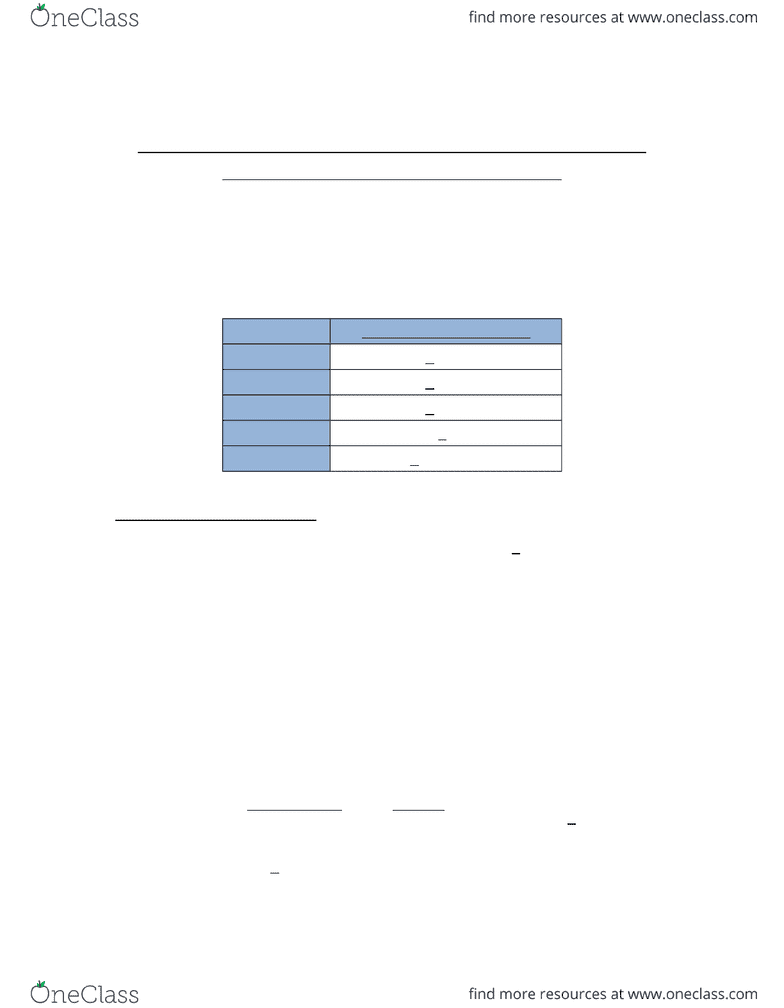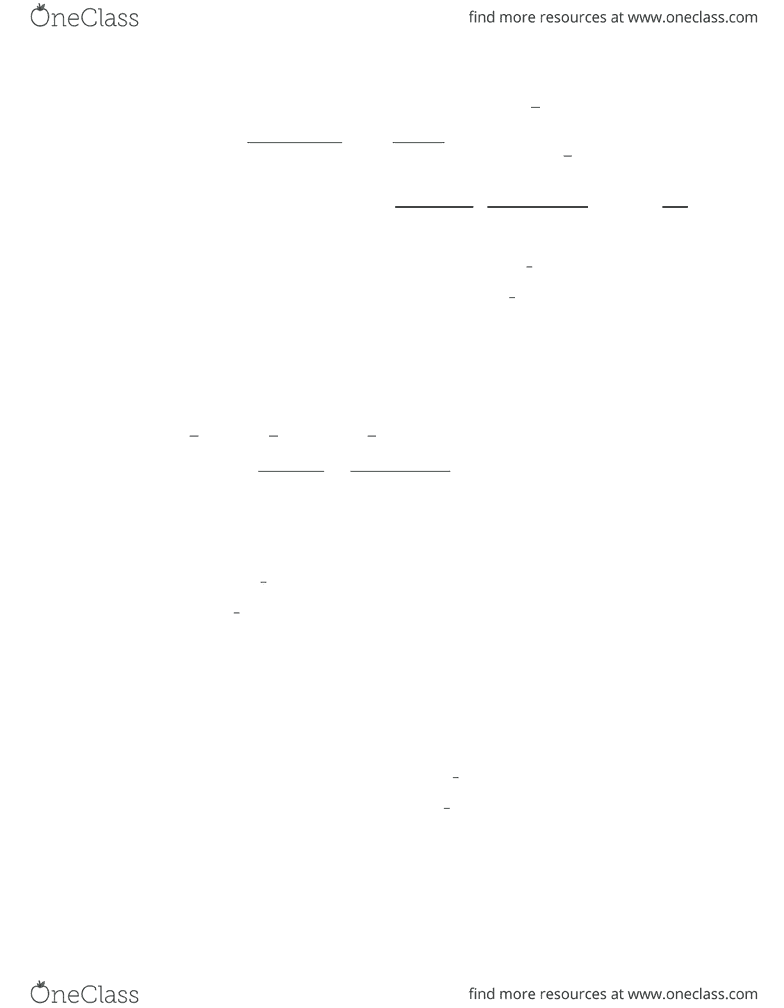Study Guides (380,000)
CA (150,000)
McGill (6,000)
Chemistry (200)
Quiz

# CHEM 297 Study Guide - Quiz Guide: Volumetric Flask, Potassium Thiocyanate, Silver Nitrate

Department
Chemistry
Course Code
CHEM 297
Professor
Jan Hamier
Study Guide
Quiz

This preview shows pages 1-2. to view the full 6 pages of the document.Has My Milk Been Watered Down? An Assessment via the
Determination of it’s Chloride Content
Name: Lab Day: Friday
ID #: Date: Wednesday
November 18
Locker #: 115 TA: Xiying
Final Experimental Value
Replicate 1 1.946 + 0.0058 g/L
Replicate 2 1.842 + 0.0055 g/L
Replicate 3 1.828 + 0.0054 g/L
Average 1.872 g/L + 0.0060 g/L
Std.Dev (S) + 0.064 g/L
I – Propagation of Uncertainty
Given that the random uncertainty of the analytical balance was + 0.00005 g,
e1
2+e2
2
¿
¿
¿
(0.000052+0.000052)1/2=±0.00007
Uncertainty of Mass of Powdered Milk
Massnet = Massinitial – Massfinal = 29.4615 g - 27.2099 g = 2.2516 g
±0.00007
Percentage Error =
Absolute Error
Reading ×100=0.00007
2.2516 ×100
= 2.2516 g + 0.000031%
Mass of milk = 2.2516 g + 0.000031%
Uncertainty of Concentration of AgNO3

Only pages 1-2 are available for preview. Some parts have been intentionally blurred.MassAgNO3 = Massinitial – Massfinal = 32.3902 g - 23.9048 g = 8.4854 g + 0.00007
Percentage Error =
Absolute Error
Reading ×100=0.00007
8.4854 ×100
= 8.4854g + 0.00082%
[
AgN O3
]
=Mas sAgNO 3× Molar Mas sAgNO3×1
Volum edilution
=8.4854 ×169.87
0.05 =0.099905 mol
L
Percentage Error of Concentration of AgNO3:
0.0008 22+0.0322¿
1
2=0.0320
e1
2+e2
2¿
1
2=¿
eTotal=¿
Uncertainty of Normality of KSCN
Given that the random uncertainty of the volumetric flask, the 25 mL pipette and the
burette was + 0.113 mL, + 0.03 mL, and + 0.1 mL respectively
[AgN O3]×0.025 L × 1
Liter sKSCN
×=0.09991×0.025
0.02107 =0.1185 N
Normality of KSCN =¿
Mean Normality = (Normality1 x Normality2 x Normality3)/3 = 0.1175 N
0. 12+0.03202+0.152¿
1
2=0.1831
e1
2+e2
2¿
1
2=¿
eTotal=¿
Uncertainty of Mass of Chlorine in Liters of Milk
Mas sChlorine=
(
[
AgN O3
]
Volum eAgNo 3
[
KSCN
]
Volum eKSCN
)
× Molar MassChlorine=
(
0.099905×0.05000.1185 ×34.19
)
×35.453=0.03464 g
Percentage Error for mol of AgNO3:
###### You're Reading a Preview

Unlock to view full version